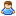1.This forum is obsolete and read-only. Feel free to contact us at support.keenswh.com

# [Guide] Programmable Block - C# 103 for Space Engineers - Math Class

Discussion in 'Programming Guides and Tools' started by Textor, Jan 7, 2015.

This last post in this thread was made more than 31 days old.
1. ### TextorJunior Engineer

Messages:
775
This guide assumes you have read C# 101 and 102. This also assumes you know how to add, subtract, multiply, and divide, and some basic algebra (if not higher). If you do not know how to do these things, please see your local math teacher.

So, why math? Because this is programming, that's why! Computers compute numbers. If you don't know how to manipulate numbers, you won't get far in coding, C# or otherwise.

Order of Operations
So, let's start at the very basics: PEMDAS. Many people remember it from various mnemonics, such as "Please Excuse My Dear Aunt Sally" (I don't need to see examples of how you learned this, that isn't the point here.)

Order of Operations is very important to computers. So important that they enforce them. So:

Parentheses are always evaluated first.
Exponents are next.
Multiplication is next.
Division after that.
Subtraction after that.

(If you don't recall, (* and /), and (+ and -) are interchangeable, but used in the order they appear, from left to right).

Code:
```int i = (1+5)*(8+1); /*54*/
int j = 1 + 5 * 8 + 1; /*42*/
int k = 1+ 5 * (int)Math.Pow(2.0,3.0) + 1; /*42*/
int l = (1+5) * (int)Math.Pow(2.0,3.0) + 1; /*49*/
```
Zero, Dividing By

DON'T. EVER. At best, you'll get ERR: DIV#0 (or NaN). At worst it'll refuse to run. If you are dividing by a number that might possibly be zero at any point always add a check to make sure that it is more than zero first.Why? Well, solve this equation:

1/0 = ?

When you do, get back to me, and contact the Nobel committee while you're at it.

Math Library
Ok, so, enough of that. What's this weird "Math.Pow" thing?

Well, C# doesn't have an operator for exponents, so you get Math.Pow():

Code:
```double Math.Pow(double x, double y)
```
For those who aren't familiar, this is the equivalent to: x^y.

Math also has a lot of other functions to use. I will not explain when to use these-- you will need to know this yourself. NOTE: Almost everything here either uses the double or decimal type.

Abs() - Absolute Value (all types)
Acos() - Return the angle of the cosine.
Asin() - same as above, only sin
Atan() - Same as above, only tangent.
Atan2() - Return the angle that is the tangent of two values
Ceiling() - Return the next integer up from your current decimal (1.01 = 2)
Floor() - Return the next integer down from your current decimal (1.9 = 1)
Cos() - cosine
Cosh() - Hyperbolic cosine
Exp() - Returns e^x
Log(x) - Logarithm (base e)
Log(x, y) - Logarithm (base y)
Log10() - Logarithm (base 10)
Max(x,y) - Returns the larger number (compatible types only) (all types)
Min(x,y) - Returns the smaller number (compatible types only) (all types)
Round() - rounds the number to the nearest integer. (1.01 = 1. 1.9 = 2)
Round(x,y) - Round the number to the specified precision (x = decimal/double, y = int). So Round(1.024,2) = 1.02
Sign() - Checks positive/negative, returns a number: -1 (negative), 0 (value is 0), 1 (positive) - (all types)
Sin() - Sine
Sinh() - Hyperbolic Sine
Sqrt() - Square Root
Tan() - Tangent
Truncate() - Cuts the number off at the decimal point. Basically, casts it to int and returns the value back as the number.0: Truncate(1.01) = 1.0

Math.E - e, the constant.
Math.PI - Pi, the constant.

Last edited: Apr 13, 2015
•Like x 4
2. ### LurchTrainee Engineer

Messages:
27
Good guide Textor, I may slowly be coming to grips with this.